﻿ New Technique to Determination of Electronic Circuits for Semiconductor Components by Recognizing Nyquist Curve

### New Technique to Determination of Electronic Circuits for Semiconductor Components by Recognizing Ny...

El Mahdi Barrah, Rachid Ahdid, Said Safi, Abdessamad Malaoui

Journal of Computer Sciences and Applications

## New Technique to Determination of Electronic Circuits for Semiconductor Components by Recognizing Nyquist Curve

El Mahdi Barrah1, Rachid Ahdid1, Said Safi1, Abdessamad Malaoui1,1Interdisciplinary Laboratory of Research in Sciences and Technologies (LIRST), Sultan Moulay Slimane University, Béni Mellal, Morocco

### Abstract

In this paper, we present an automatic recognition system of electronic components. The recognition system is based on digital image of Nyquist curves drawn by transfer functions of their equivalent impedances. In the first step, we construct a database of Nyquist diagram of 17 known circuits. In the second step, we apply shape analysis algorithms of curves such as: Fourier Descriptors (FD), Freeman Coding (FC) and Hu Moments (HM). In classification part, we use the Neural Networks (NN). The simulation results demonstrates that the proposed method give a good results.

• El Mahdi Barrah, Rachid Ahdid, Said Safi, Abdessamad Malaoui. New Technique to Determination of Electronic Circuits for Semiconductor Components by Recognizing Nyquist Curve. Journal of Computer Sciences and Applications. Vol. 3, No. 4, 2015, pp 100-104. http://pubs.sciepub.com/jcsa/3/4/3
• Barrah, El Mahdi, et al. "New Technique to Determination of Electronic Circuits for Semiconductor Components by Recognizing Nyquist Curve." Journal of Computer Sciences and Applications 3.4 (2015): 100-104.
• Barrah, E. M. , Ahdid, R. , Safi, S. , & Malaoui, A. (2015). New Technique to Determination of Electronic Circuits for Semiconductor Components by Recognizing Nyquist Curve. Journal of Computer Sciences and Applications, 3(4), 100-104.
• Barrah, El Mahdi, Rachid Ahdid, Said Safi, and Abdessamad Malaoui. "New Technique to Determination of Electronic Circuits for Semiconductor Components by Recognizing Nyquist Curve." Journal of Computer Sciences and Applications 3, no. 4 (2015): 100-104.

 Import into BibTeX Import into EndNote Import into RefMan Import into RefWorks

1234
Prev Next

### 1. Introduction

Nowadays, passing algorithms of an electronic circuit (transfer function) to their Nyquist plot in the literature have reached a decent level of performance, but the problem is finding the equivalent circuit from a Nyquist diagram found by an electronic component .

To identify the problem, we conducted studies at deferent levels of recognition chain. These studies concern the deferent methods and preprocessing algorithms, normalization...etc. To construct a database of well-known electronic circuits and their Nyquist diagrams. Also as learning and recognition strategies based on local and global approaches.

The aim of our work is build an automatic recognition system of electronics circuit, for this we construct a database of Nyquist diagrams of the for various known electronic circuits and compare them automatically with the Nyquist diagram of the electronic component test using the preprocessing algorithms, feature extraction and classification.

This paper is organized as follows: In section two, we present the basic analysis of electronic components and their Nyquist diagrams. In section three, we describe the steps to follow to build our database. In section four, we present the electronic component recognition system by analyzing the curves of Nyquist. In the last section we present the results of simulation.

### 2. Electronic Components Analysis

2.1. Electronic Circuits Single

An electronic circuit is a set of electronic components (Resistor, Capacitor, Self) often interconnected using a printed circuit board and whose purpose is to perform a function. That is why an electronic circuit is often regarded as a black box with an inlet and an outlet for food.

To achieve our recognition system it is necessary to build a database of simple circuits whose Nyquist plots are known.

2.2. Nyquist Diagram

The Nyquist diagram is the most immediate representation of the complex frequency response function: reported in the plan the real part along the horizontal axis and the imaginary part along the vertical axis [1, 2, 3, 4, 15].

After selecting circuits our database, we need to plot their Nyquist diagrams for this it is necessary to determine the transfer function which is equivalent to the electronic circuit impedance study. In Figure 1 we represent four examples of Nyquist diagrams.Download asVeiw figureFigures index
Figure 1. RLC series model
2.3. Electrical Circuits

The most classic circuit in literature is the RLC circuit. Due to its great usefulness in the study of linear systems, the model of the RLC circuit helps to understand some of the behaviors of an electrical control system. Thus, the next subsections will address to the modeling of combined in series and parallel RLC circuits.

Model I: Resistance, capacitance and inductance in series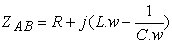(1)

The Figure 1 shown a RLC series, this model can be used to represent any positive or negative phase. Other simple circuits are products from this circuit playing on the values of C, R and L.

For example, the advanced phase circuit is obtained when C = 0:(2)

Also the delayed phase circuit is obtained when L = 0: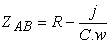(3)

Model II: Randles circuitDownload asVeiw figureFigures index
Figure 2. Randles model

The randle circuit is one of the simplest and the most common cell models. It includes a solution resistance, a double layer capacitor and a polarization resistance.

As shown in Figure 2. The impedance is given by the relationship: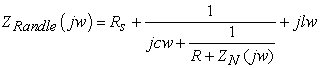(4)

With the Nernst’s static impedance is given by:(5)is the Nernst’s static resistance D is the diffusion coefficient and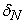is the diffusion’s length

Model III: RLC-Warburg circuitDownload asVeiw figureFigures index
Figure 3. RCL-Warburg model

This model’s impedance is given by the relation: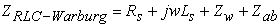(6)(7)Download asVeiw figureFigures index
Figure 4. Examples of Electronic Circuits and their Nyquist diagrams

From these four circuits, the other models of inferior order may be deduced by taking the limit values of R, L, or C (0 or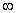). The transfer functions of these electric models are given by:(8)

Where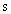is the Laplace variable (). To simplify the numerical study of these elementary models, some approximations have been used.

### 3. Electronic Components Syste

3.1. Building the Database

To construct our database we have to analyze electronically the 17 circuits: first, we determine their transfer functions (equivalent impedances) and plot their Nyquist diagrams. Second, we compute image processing of Nyquist curves. This computer study is based on the following pretreatment steps: binarization, histogram equalization, standardization and save the images in a selected extension (jpg, png, bpm, ...).

The following diagram summarizes the steps to follow to achieve our database:Download asVeiw figureFigures index
Figure 5. Principle Steps of Constructing Our DatabaseDownload asVeiw figureFigures index
Figure 6. Electronic Components Recognition System
3.3. Pretreatment

The preprocessing step is the first task of a recognition system. This step is not specific to the recognition of electronic components but part of any recognition system. In this step we use the techniques of image processing to improve the perception of filtering image noise or reducing the amount of information to be processed to keep only the most relevant information. In this paper we have followed three pretreatment steps: normalization, histogram equalization and binarization.Download asVeiw figureFigures index
Figure 7. Principle steps of preprocessing
3.4. Extracting Features

To analyze the shape of the Nyquist curves characterizing the circuits of our database, we propose three methods: Fourier descriptors, Freeman coding and Hu moments.

Fourier Descriptors (FD):

Fourier descriptors are a mathematical tools used to describe the outer shell a shape that is to say its contour. There are two types of Fourier Descriptor: descriptor by tangent is used to describe continuous contours and complex descriptors are used to describe discrete contours. Given that the study concern digital images represented by a pixel list. So we chose to use the complex descriptors [5, 6, 7]. In this representation, the shape has been described by a set of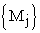points and it was represented in the complex plane. At each point of the contour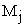was therefore attached a complex number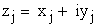whereandrepresentedpoint coordinates in the coordinate system of the image.

Then Fourier descriptor coefficients of the Fourier transform of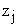is described by the: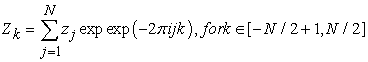(9)

A Fourier descriptor could be seen as a reversible operator to switch between the image space in Fourier space, and from a spectrum in Fourier space, we could perfectly reconstruct a discrete contour shape in the image space.

●, Z represent the position of center gravity of shape

●, except for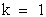, circle shape of radius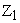(Z1 is a scale factor; if standardization, invariant shape by scaling)

●  Factorsand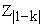: roles opposite but symmetrical on the curve.

The order k is the number of shares on the unit circle (between 0 and):

●  1 Action for k = 2 and k = - 1,

●  2 Action for k = 3 and k = - 2,

●  3 Action for k = 4 and k = - 3,

●  etc.

More coefficients are numerous more contour is complex. In addition, the more the values of the coefficients are high for more details are on the curve.

In the discrete domain, descriptors are replaced by a finite set of values forming the components of a vector that can be used at the input of a classifier.

Hu Moments (HM):

The central moments of f (x, y) are defined as: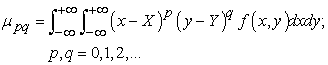(10)

Where, the pixel point (X, Y) are the centroid of the image f(x,y).(11)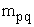is a moment sequence of the image function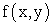, are defined as: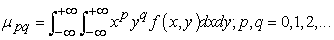(12)

The momentscomputed using the centroid of the imageis equivalent to thewhose center has been shifted to centroid of the image. Therefore, the central moments are invariant to image translations. Scale invariance can be obtained by normalization. The normalized central moments are defined as follows: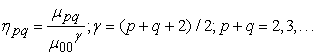(13)

The method of invariants moment is derived from algebraic invariants applied to the moment generating function under a rotation transformation. The set of absolute invariants moment consists of a set of nonlinear combinations of central moments that remain invariant under rotation. Hu defines the following seven functions computed from central moments through order three that are invariant with respect to object scale translation and rotation: [8, 9, 10].

Ø1 = η20 + η02

Ø2 = (η20 - η02)2 + 4 η211

Ø3 = (η30 - η12)2 + (η21 - η03)2

Ø4 = (η30 + η12)2 + (η21 + η03)2

Ø5 = (η30 - 3 η12)(η30 + η12)[(η30 + η12)2 - 3(η21 + η03)2] + (3η21 - η03)(η21 + η03)[3(η30 + η12)2 - (η21 + η03)]

Ø6 = (η20 - η02)[(η30 + η12)2 - (η21 + η03)2 ] + 4η1130 + η12)(η21 + η03)]

Ø7 = (3η21 – η03)(η30 + η12)[(η30 + η12)2 - 3(η21 + η03)2] - (η30 - η12)(η21 + η03)[3(η30 + η12)2 - (η21 + η03)2]

The Freeman Code (FC):

In dimension 2, on a square grid, one can study the properties of objects using the neighborhood four V4 noted in (i, j) or neighborhood eight points noted V8 (i, j) of a pixel (i, j) where i and j represent the row number and the column number of the pixel in the image. These neighborhoods are represented in Figure 8. They correspond to four and eight pixels closest to the pixel (i, j) [11, 12].Download asVeiw figureFigures index
Figure 8. Neighborhoods V4 (left) and V8 (right) of a pixel (i, j), i and j respectively represent the row number and the column number

Freeman code, first introduced in 1961 [11, 12], is to encode the contour of an object by:

• Absolute information corresponding to the coordinates of a starting point.

• A chain coding giving the relative position of the next point of the contour of the object according to one of the representations shown in Figure 8 (encoding using 4 or 8 directions). Thus, using 8 directions, the encoding of 0 means that the next pixel of the contour of the object is to the right of the current pixel, and the encoding means 5 according to a pixel at the bottom left of the current pixel.

Each of the 4 and 8 directions can be respectively coded on 2 and 3 bits, leading to a high compression without loss of the image.

Neural Network (NN):

The neural network is used here to learn the information contained in the Hu Moments (HM), Freeman Code (FC) and Fourier Descriptors (FD) and their relations with the curve contour. It should be noted here that makes no assumptions about the likely shape of the desired curve even that we did to the no pretreatment image .

A neural network consists of a set of interconnected neural then giving rise to networks with varying structures. For our application, we use the layers structure (Multi-Layer Perceptron: MLP). Such structure (Figure 9) disseminates information from the input layer, composed by the neural receiving primitive information to the output layer, which contains the final neurons transmitting output information processed by the entire network, while traversing a or more intermediate layers, called hidden layers. The network is well established a nonlinear system that combines, input feature vectors, the outline of curve of the output layer.Download asVeiw figureFigures index
Figure 9. Example of Multilayer neural network

### 4. Simulation Results

In this section we make a series of simulation to evaluate the effectiveness of the proposed approach. These results were performed based on data flow. This database contains 170 images of 17 Nyquist diagrams of known circuits. For each circuit, we have 10 different images of Nyquist diagrams binarized and standardized at a resolution of 283 x 390 pixels.

In our simulation, 85 Nyquist images base circuit and 85 nyquist images were used for testing the identification performance. Some results on the number of images are listed in the Table 1.

All images of Nyquist diagrams of 17 electronic circuits construct our electronic components database are shown in Figure 10:Download asVeiw figureFigures index
Figure 10. Nyquist diagrams of 17 electronic circuits construct our electronic components database

The following Table 1 summarizes the experimental results of our simulation. This table summarizes the simulation results obtained by the propsed approach. This table shows the recognition rate and the error rate found by the three methods used in analysis of Nyquist diagram: Hu Moments (HM), Freeman Code (FC) and Fourier Descriptors (FD).

When we increase the total number of training images (number of class) we see that the recognition rate by decrease but the error rate increases.

#### Table 1. Resultants of trained of our recognition databaseDownload asPowerPoint Slide

Veiw figure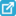View current table in a new window

The results obtained by our electronic component recognition system shows that this method is interest to resolve this problem determined the equivalent electronic circuit by the Nyquist plot of use.

### 5. Conclusion

In this paper we have described an automatic electronic circuit recognition system based on the Niquist Diagram, using tree features extraction methods such as: Fourier Descriptors (FD), Freeman Coding (FC) and Hu Moments (HM). In classification part, we used the Neural Networks (NN). The simulation results the Niquist diagram gives us o good rate (82.12%) using the Fourier Descriptors (FD).

### References

  Abdessamad Malaoui, et al. “A new approach of a precise electric modeling of the semiconductors and dielectric samples.” The IEEE International Conference on Microelectronics, pp 226-229, Marrakech, (2009).In article  E. Barsoukov, J. Ross Macdonald, “Impedance Spectroscopy Theory, John Wiley & Sons”, Inc., New Jersey, 2005.In article  N. Rizoug. Thèse de Doctorat, l’Ecole Centrale de Lille et l’Université des Sciences et Technologies de Lille, 2006.In article  S. Skales, V. Dolecek, M. Slemnik. Corrosion Science Journal, 49, No3, pp. 1045-1055, 2007.In article View Article  Laurent FANTON thèse doctorat de université de Toulouse. “Estimation de l'âge au décès à partir de la 4ième côte”; Université Toulouse III - Paul Sabatier; 8 juillet 2009.In article  N. Ben messaoud and N. Boughanemi “Reconnaissance de Caryotype Humain Par Descripteur de Fourier”; 3èmes Journées Internationales sur l’Informatique Graphique ; JIG’2007; pp : 62-68.In article  José Mennesson, Christophe Saint-Jean, Laurent Mascarilla “Un nouvel ensemble de descripteurs de Fourier Clifford pour les images couleur : les GCFD3”; HAL Id: hal-00656528 ; Lyon 24-27 Jan 2012.In article  Simon Xinmeng Liao; thèse doctorat: “Image Analysis by Moments”; Department of Electrical and Computer Engineering; The University of Manitoba Winnipeg; Manitoba Canada; 1993.In article  Zhihu Huang and Jinsong Leng. “Analysis of Hu's Moment Invariants on Image Scaling and Rotation”; Proceedings of 2nd International Conference on Computer Engineering and Technology (ICCET). (pp: 476-480). Chengdu, China. IEEE. 2010.In article  S. Conseil; S. Bourennane and L. Martin: “Comparison of fourier descriptors and hu moments for hand posture recognition’; European Signal Processing Conference (EUSIPCO) 2007.In article  H.Freeman: “On the encoding of arbitrary geometric configurations”, IRE Trans.Electron. Comput.EC-10, 260-268, 1961.In article View Article  Freeman H.: “Computer Processing of Line-Drawing Images”, Computing surveys, Vol 6, No 1, March 1974.In article View Article  V. N. Vapnik, “The Nature of Statistical Learning Theory”, 2nd ed., Springer-Verlag New York Inc., 314 pages, 2000.In article View Article  A. Crosnier, G. Abba, B. Jouvencel and R. Zapata. Ellipses Edition, Paris, 2001.In article  A. Malaoui, M. Mabrouki, M. Ankrim. “A new precise method of electric modeling of thin film”. M. J. CONDENSED MATTER, Vol 12, N°1, 2010.In article